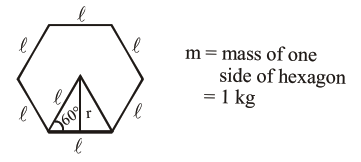# A uniform thin bar

Question:

A uniform thin bar of mass $6 \mathrm{~kg}$ and length $2.4$ meter is bent to make an equilateral hexagon. The moment of inertia about an axis passing through the centre of mass and perpendicular to the plane of hexagon is _____________ $\times 10^{-1} \mathrm{~kg} \mathrm{~m}^{2}$.

Solution:$61=2.4 \ell=0.4 \mathrm{~m}$

$\sin 60^{\circ}=\frac{r}{\ell}$

$r=1 \sin 60^{\circ}=\frac{\ell \sqrt{3}}{2}$

MOI, $\quad \mathrm{I}=\left[\frac{\mathrm{m} \ell^{2}}{12}+\mathrm{mr}^{2}\right] 6$

$=\left[\frac{\mathrm{m} \ell^{2}}{12}+\mathrm{m}\left(\frac{\ell \sqrt{3}}{2}\right)^{2}\right] 6$

$=5 \mathrm{ml}]^{2}$

$=5 \times 1 \times 0.16$

$=0.8$

$\mathrm{I}=8 \times 10^{-1} \mathrm{~kg} \mathrm{~m}^{2}$

Ans. 8